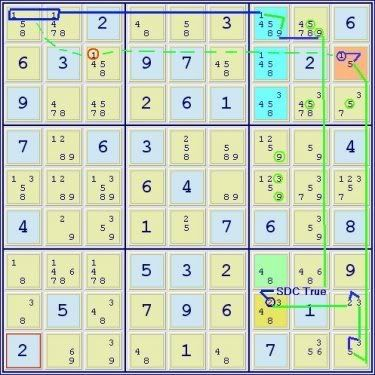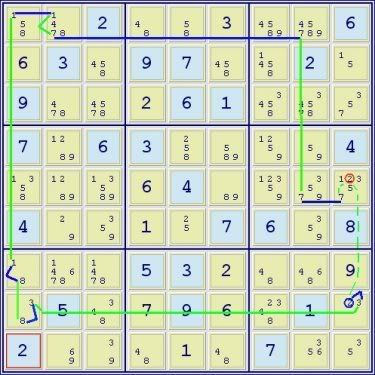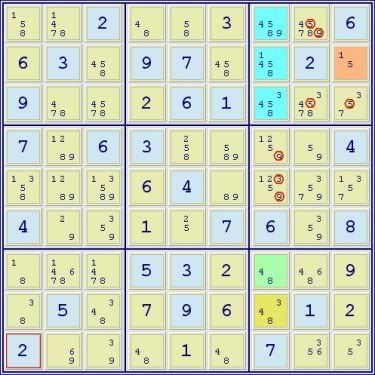## Solving with Almost Sue De Coq: Extreme #122 (ASI#2d)

Advanced methods and approaches for solving Sudoku puzzles

### Solving with Almost Sue De Coq: Extreme #122 (ASI#2d)

This is a continuation/followup of the thread at:
http://forum.enjoysudoku.com/viewtopic.php?t=6563&sid=b76053b73269ac68ba9399c670c7dc08

In this solution of Extreme #122, what is particularly interesting is that the opening chain using an aSDC, provides an elimination which makes possible the directly following chain which provides the elimination which exposes the full SDC. All of the chains which follow the SDC and, of course, solve the puzzle depend directly or indirectly on the eliminations produced by the full SDC pattern. (As usual, this is only one of what are several possible solutions.)

Extreme #122 ER=7.3 SSTS position:

Code: Select all
`*--------------------------------------------------------------------* | 158    1478   2      | 48     58     3      | 14589  45789  6      | | 6      3      1458   | 9      7      458    | 1458   2      15     | | 9      478    4578   | 2      6      1      | 3458   34578  357    | |----------------------+----------------------+----------------------| | 7      1289   6      | 3      258    589    | 1259   59     4      | | 1358   1289   13589  | 6      4      89     | 12359  3579   12357  | | 4      29     359    | 1      25     7      | 6      359    8      | |----------------------+----------------------+----------------------| | 18     14678  1478   | 5      3      2      | 48     468    9      | | 38     5      348    | 7      9      6      | 2348   1      23     | | 2      69     39     | 48     1      48     | 7      356    35     | *--------------------------------------------------------------------*`The core of the SDC in r123c7 is in blue, the A cell is in brown at r2c9, the B cell is in green at r7c7 and since this is an aaals core with 6 digit values in 3 squares, a C cell is necessary & is present in gold at r8c7 to complete the pattern. But for the 2 at r8c7 this would be a full SDC -the digits circled in green are the eliminations that would be possible from the full pattern:

(1=5)r2c9 - (5=3)r9c9 - (3=2)r8c9 - (2)r8c7 = SDC(134589)r123c7/(15)r2c9/(48)r7c7/(348)r8c7 - (9)r1c8 = (9-1)r1c7 = grp(1)r1c12
=> r2c3<>1 -> linebox(1)r2c79:r1c7<>1
NL: r2c3 -1- r2c9 -5- r9c9 -3- r8c9 -2- r8c7 =2= SDC(134589)r123c7/(15)r2c9/(48)r7c7/(348)r8c7 -9- r1c8 =9= r1c7 =1= r1c12 -1- r2c3 => r2c3<>1 -> linebox(1)r2c79:r1c7<>1Red highlights the r8c7<>2 elimination which exposes the full SDC.

(2=3)r8c9 - (3=8)r8c1 - (8=1)r7c1 - r1c1 = (1-7)r1c2 = r1c8 - r5c8 = (7)r5c9 => r5c9<>2, r8c9=2, r8c7<>2

NL: r5c9 =7= r5c8 -7- r1c8 =7= r1c2 =1= r1c1 -1- r7c1 -8- r8c1 -3- r8c9 -2- r5c9 => r5c9<>2, r8c9=2, r8c7<>2SDC(134589)r123c7/(15)r2c9/(48)r7c7/(348)r8c7 => r3c89<>5, r1c8<>59, r45c7<>9, r5c7<>3 -> r1c7=9

Final chains which complete the solution:

(3=7)r3c9 - r1c8 = (7-1)r1c2 = r1c1 - (1=8)r7c1 - (8=3)r8c1 - r8c7 = r3c7 - loop => r1c2<>48, r3c8<>37, r8c3<>3
NL: r1c8 =7= r1c2 =1= r1c1 -1- r7c1 -8- r8c2 -3- r8c7 =3= r3c7 -3- r3c9 -7- r1c8 => r1c2<>48, r3c8<>37, r8c3<>3

(4)r2c6 = xw(4)r28c37 - (4=8)r7c7 - (8=1)r7c1 - r1c1 = (1-7)r1c2 = r1c8 - (7=3)r3c9 - r3c7 = r8c7 - grp(3)r9c89 = (3-9)r9c3 = r9c2 - (9=2)r6c2 - (2=5)r6c5 - r1c5 = (5)r2c6 - loop
=>r45c2<>9, r4c5<>5, r7c238<>8 -> linebox(8)r78c7:r23c7<>8
NL: r2c6 =4= xw(4)r28c37 -4- r7c7 -8- r7c1 -1- r1c1 =1= r1c2 =7= r1c8 -7- r3c9 -3- r3c7 =3= r8c7 -3- r9c89 =3= r9c3 =9= r9c2 -9- r6c2 -2- r6c5 -5- r1c5 =5= r2c6 =>r45c2<>9, r4c5<>5, r7c238<>8 -> linebox(8)r78c7:r23c7<>8

(4)r3c2 = r7c2 - (4=8)r8c3 - grp(8)r78c1 = hp(58-1)r15c1 = (1-7)r1c2 = (7-8)r1c8 = (8)r3c8 => r3c3<>4=8
NL: r3c8 -4- r3c2 =4= r7c2 -4- r8c3 -8- r78c1 =8= hp(58)r15c1 -1- r1c1 =1= r1c2 =7= r1c8 =8= r3c8 => r3c3<>4=8

(2=5)r6c5 - (5=8)r1c5 - (8=4)r1c4 - r1c8 = grp(4)r23c7 - (4=8)r7c7 - (8=1)r7c1 - r7c3 = r5c3 - als(1=28)r45c2
=> r6c2<>2=9, r9c2=6, r7c8=6, r6c5=2 etc.
NL: r6c2 -2- r6c5 -5- r1c5 -8- r1c4 -4- r1c8 =4= r23c7 -4- r7c7 -8- r7c1 -1- r7c3 =1= r5c3 -1- als(28)r45c2 -2- r6c2 => r6c2<>2=9, r9c2=6, r7c8=6, r6c5=2 etc.

Singles to the End
DonM
2013 Supporter

Posts: 475
Joined: 13 January 2008

### Re: Solving with Almost Sue De Coq: Extreme #122 (ASI#2d)

Extreme #122 ER=7.3 SSTS position:

Code: Select all
`*--------------------------------------------------------------------* | 158    1478   2      | 48     58     3      | 14589  45789  6      | | 6      3      1458   | 9      7      458    | 1458   2      15     | | 9      478    4578   | 2      6      1      | 3458   34578  357    | |----------------------+----------------------+----------------------| | 7      1289   6      | 3      258    589    | 1259   59     4      | | 1358   1289   13589  | 6      4      89     | 12359  3579   12357  | | 4      29     359    | 1      25     7      | 6      359    8      | |----------------------+----------------------+----------------------| | 18     14678  1478   | 5      3      2      | 48     468    9      | | 38     5      348    | 7      9      6      | 2348   1      23     | | 2      69     39     | 48     1      48     | 7      356    35     | *--------------------------------------------------------------------*`

Don
An alternative using one initial move which virtually takes care of the puzzle : looks worse than it is :
the idea is to exploit the hidden triple 128r456c2.
Each of the lines A, B, C that follow pick up the position at the end of move 1.
Note : this is not a net, simply three different routes that each produce eliminations
1. 128r456c2=9r456c2-(9=6)r9c2-6r9c8=3579r4569c8-3579r13c8
A=7r3c9-7r3c23=7r1c2 : =><18>r1c2
B=48r1c8-(8=5)r1c5-(58=1)r1c1-(1=8)r7c1 : =><18>r5c1
C
(i)=48r3c8
(ii)=7r3c9
-(487=5)r3c3-(5=39)r6c3-(3=48)r8c3
(iii)=15r2c79-(15=48)r2c3
:=><8>r5c3.
Therefore
=>pointing 8s r45c2 : <8>r37c2
=>47r13c2 : <47>r3c3 <47>r8c2 <4>r3c2
=>placement 7 r7c3
=>placement 4r8c3
2. (1=5)r2c9-(5=3)r9c9-(3=9)r9c3-(9=6)r9c2-(6=1)r7c3-1r45c2=1r5c3 : =><1>r3c2 : multiple placements.
aran

Posts: 334
Joined: 02 March 2007

Aran, very interesting- I've noticed that you've made good use of hidden triples in other solutions you've posted. I still don't make use of them as much as I'd like to. Seeing alternative manual solutions like this makes these threads that much more useful.
DonM
2013 Supporter

Posts: 475
Joined: 13 January 2008